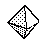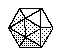Name: ___________________Date:___________________

 Email us to get an instant 20% discount on highly effective K-12 Math & English kwizNET Programs!

### Grade 3 - Mathematics8.14 Regular Polyhedrons

 A prism is a three dimensional figure with two same, parallel bases that are polygons. A 3 dimensional shape with all equal faces is called a polyhedron. A polyhedron having four faces is called tetrahedron. Polyhedrons are named after the number of faces they have. They are prefixed as tetra-4, penta-5, hexa -6, hepta- 7, octa-8, nona -9, deca- 10, 12-dodeca, 20-icosa. Directions: Answer the following questions. Also draw at least five examples of regular polyhedrons of your own.Name: ___________________Date:___________________

### Grade 3 - Mathematics8.14 Regular Polyhedrons

 Q 1: What is the shape with 8 faces, each formed by an equilateral triangle?pentahedronoctahedronhexahedron Q 2: What is the shape with 20 faces, each formed by an equilateral triangle?hydronicosahedrontwentyhedron Q 3: A 3 dimensional shape with all faces of the same shape and same size is called aPolyhedronPentagonPolygon Q 4: What is the shape with 12 faces, each formed by an regular pentagon?dodecahedron12hedronsdocahedron Question 5: This question is available to subscribers only! Question 6: This question is available to subscribers only!